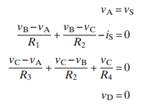Create an Account

Home / Questions / The following are a set of node-voltage equations; draw the circuit they represent.

The following are a set of node-voltage equations; draw the circuit they represent.

The following are a set of node-voltage equations; draw the circuit they represent.Jul 28 2020 View more View LessSubscribe To Get Solution